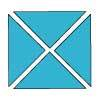#### You may also like### Chocolate

There are three tables in a room with blocks of chocolate on each. Where would be the best place for each child in the class to sit if they came in one at a time?### Four Triangles Puzzle

Cut four triangles from a square as shown in the picture. How many different shapes can you make by fitting the four triangles back together?### Cut it Out

Can you dissect an equilateral triangle into 6 smaller ones? What number of smaller equilateral triangles is it NOT possible to dissect a larger equilateral triangle into?

# Carrying Cards

##### Age 7 to 11Challenge Level

A Maths Club at Beacon School Amersham wrote:

First we worked out all the missing numbers for the red, blue and yellow children. This was easy because we were told how a number could be made from the number on the card before it in the same row.
Then we spotted that the children in green shirts have a number which is the sum of the yellow and blue shirts in the same row.
We then worked out the fifth row by looking for a pattern. The red shirt numbers went up in ones, the blue shirt numbers went up in ones, the yellow shirt numbers went up in 2s and the green shirts went up in 3s.

Well done! They sent in a table to represent the children's numbers:

 5 9 10 19 4 8 8 16 3 7 6 13 2 6 4 10 1 5 2 7

Fantastic solutions were also sent in by lots of pupils from Crosshall Junior School; Jun and Colin from the Canadian Academy; Karnan from Stag Lane Middle School; Richard and Jacob from St Thomas More's School and Ellie from West Bridgford Juniors.

Trang from Central Foundation Girls' School, used symbols to help her write out what she needed to do:

First, I wrote the short letter for the colours: Red: $r$ ; Blue: $b$ ; Yellow: $y$; Green: $g$
Then I wrote out the fomula: $b = r + 4y = 2r$
Therefore: For the 1st row I have: $y = 4 \times 2 = 8$;
2nd row: $r = 6 \div 2 = 3$; $b = 3 + 4 = 7$
3rd row: $r = 4 \div 2 = 2$
4th row: $r = 2\div2 = 1$
Looking at the first and the last rows, I noticed that the blue and the yellow in the same rows added together equal the green.
So green in the 2nd row $= 7 + 6 = 13$ and the 3rd row $= 4 + 6 = 10$
I also noticed that the numbers in the red column are consecutive numbers ($1, 2, 3, 4$). It's also similar to the blue column ($5, 6, 7, 8$) and the yellow column is the multiples of $2$ ($2, 4, 6, 8$). So if there is one more row - the red colour and the blue colour need to add $1$ for each and the yellow need to times $2$ and the green is made by adding the blue and yellow.